# 3.1 Complex numbers  (Page 4/8)

 Page 4 / 8

## Dividing complex numbers

Divide $\text{\hspace{0.17em}}\left(2+5i\right)\text{\hspace{0.17em}}$ by $\text{\hspace{0.17em}}\left(4-i\right).$

We begin by writing the problem as a fraction.

$\frac{\left(2+5i\right)}{\left(4-i\right)}$

Then we multiply the numerator and denominator by the complex conjugate of the denominator.

$\frac{\left(2+5i\right)}{\left(4-i\right)}\cdot \frac{\left(4+i\right)}{\left(4+i\right)}$

To multiply two complex numbers, we expand the product as we would with polynomials (the process commonly called FOIL).

Note that this expresses the quotient in standard form.

## Substituting a complex number into a polynomial function

Let $\text{\hspace{0.17em}}f\left(x\right)={x}^{2}-5x+2.\text{\hspace{0.17em}}$ Evaluate $\text{\hspace{0.17em}}f\left(3+i\right).$

Substitute $\text{\hspace{0.17em}}x=3+i\text{\hspace{0.17em}}$ into the function $\text{\hspace{0.17em}}f\left(x\right)={x}^{2}-5x+2\text{\hspace{0.17em}}$ and simplify.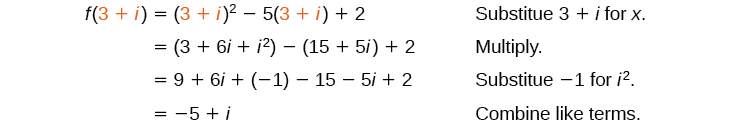Let $\text{\hspace{0.17em}}f\left(x\right)=2{x}^{2}-3x.\text{\hspace{0.17em}}$ Evaluate $\text{\hspace{0.17em}}f\left(8-i\right).$

$102-29i$

## Substituting an imaginary number in a rational function

Let $\text{\hspace{0.17em}}f\left(x\right)=\frac{2+x}{x+3}.\text{\hspace{0.17em}}$ Evaluate $\text{\hspace{0.17em}}f\left(10i\right).$

Substitute $\text{\hspace{0.17em}}x=10i\text{\hspace{0.17em}}$ and simplify.

Let $\text{\hspace{0.17em}}f\left(x\right)=\frac{x+1}{x-4}.\text{\hspace{0.17em}}$ Evaluate $\text{\hspace{0.17em}}f\left(-i\right).$

$-\frac{3}{17}+\frac{5i}{17}$

## Simplifying powers of i

The powers of $\text{\hspace{0.17em}}i\text{\hspace{0.17em}}$ are cyclic. Let’s look at what happens when we raise $\text{\hspace{0.17em}}i\text{\hspace{0.17em}}$ to increasing powers.

$\begin{array}{l}{i}^{1}=i\\ {i}^{2}=-1\\ {i}^{3}={i}^{2}\cdot i=-1\cdot i=-i\\ {i}^{4}={i}^{3}\cdot i=-i\cdot i=-{i}^{2}=-\left(-1\right)=1\\ {i}^{5}={i}^{4}\cdot i=1\cdot i=i\end{array}$

We can see that when we get to the fifth power of $\text{\hspace{0.17em}}i,\text{\hspace{0.17em}}$ it is equal to the first power. As we continue to multiply $\text{\hspace{0.17em}}i\text{\hspace{0.17em}}$ by itself for increasing powers, we will see a cycle of 4. Let’s examine the next 4 powers of $\text{\hspace{0.17em}}i.$

$\begin{array}{l}{i}^{6}={i}^{5}\cdot i=i\cdot i={i}^{2}=-1\\ {i}^{7}={i}^{6}\cdot i={i}^{2}\cdot i={i}^{3}=-i\\ {i}^{8}={i}^{7}\cdot i={i}^{3}\cdot i={i}^{4}=1\\ {i}^{9}={i}^{8}\cdot i={i}^{4}\cdot i={i}^{5}=i\end{array}$

## Simplifying powers of $\text{\hspace{0.17em}}i$

Evaluate $\text{\hspace{0.17em}}{i}^{35}.$

Since $\text{\hspace{0.17em}}{i}^{4}=1,\text{\hspace{0.17em}}$ we can simplify the problem by factoring out as many factors of $\text{\hspace{0.17em}}{i}^{4}\text{\hspace{0.17em}}$ as possible. To do so, first determine how many times 4 goes into 35: $\text{\hspace{0.17em}}35=4\cdot 8+3.$

${i}^{35}={i}^{4\cdot 8+3}={i}^{4\cdot 8}\cdot {i}^{3}={\left({i}^{4}\right)}^{8}\cdot {i}^{3}={1}^{8}\cdot {i}^{3}={i}^{3}=-i$

Can we write $\text{\hspace{0.17em}}{i}^{35}\text{\hspace{0.17em}}$ in other helpful ways?

As we saw in [link] , we reduced $\text{\hspace{0.17em}}{i}^{35}\text{\hspace{0.17em}}$ to $\text{\hspace{0.17em}}{i}^{3}\text{\hspace{0.17em}}$ by dividing the exponent by 4 and using the remainder to find the simplified form. But perhaps another factorization of $\text{\hspace{0.17em}}{i}^{35}\text{\hspace{0.17em}}$ may be more useful. [link] shows some other possible factorizations.

 Factorization of $\text{\hspace{0.17em}}{i}^{35}$ ${i}^{34}\cdot i$ ${i}^{33}\cdot {i}^{2}$ ${i}^{31}\cdot {i}^{4}$ ${i}^{19}\cdot {i}^{16}$ Reduced form ${\left({i}^{2}\right)}^{17}\cdot i$ ${i}^{33}\cdot \left(-1\right)$ ${i}^{31}\cdot 1$ ${i}^{19}\cdot {\left({i}^{4}\right)}^{4}$ Simplified form ${\left(-1\right)}^{17}\cdot i$ $-{i}^{33}$ ${i}^{31}$ ${i}^{19}$

Each of these will eventually result in the answer we obtained above but may require several more steps than our earlier method.

Access these online resources for additional instruction and practice with complex numbers.

## Key concepts

• The square root of any negative number can be written as a multiple of $\text{\hspace{0.17em}}i.\text{\hspace{0.17em}}$ See [link] .
• To plot a complex number, we use two number lines, crossed to form the complex plane. The horizontal axis is the real axis, and the vertical axis is the imaginary axis. See [link] .
• Complex numbers can be added and subtracted by combining the real parts and combining the imaginary parts. See [link] .
• Complex numbers can be multiplied and divided.
• To multiply complex numbers, distribute just as with polynomials. See [link] , [link] , and [link] .
• To divide complex numbers, multiply both the numerator and denominator by the complex conjugate of the denominator to eliminate the complex number from the denominator. See [link] , [link] , and [link] .
• The powers of $\text{\hspace{0.17em}}i\text{\hspace{0.17em}}$ are cyclic, repeating every fourth one. See [link] .

## Verbal

Explain how to add complex numbers.

Add the real parts together and the imaginary parts together.

What is the basic principle in multiplication of complex numbers?

Give an example to show the product of two imaginary numbers is not always imaginary.

$i\text{\hspace{0.17em}}$ times $\text{\hspace{0.17em}}i\text{\hspace{0.17em}}$ equals –1, which is not imaginary. (answers vary)

What is a characteristic of the plot of a real number in the complex plane?

## Algebraic

For the following exercises, evaluate the algebraic expressions.

evaluate $\text{\hspace{0.17em}}f\left(2i\right).$

$-8+2i$

evaluate $\text{\hspace{0.17em}}f\left(i\right).$

evaluate $\text{\hspace{0.17em}}f\left(2+i\right).$

$14+7i$

evaluate $\text{\hspace{0.17em}}f\left(2-3i\right).$

evaluate $\text{\hspace{0.17em}}f\left(5i\right).$

$-\frac{23}{29}+\frac{15}{29}i$

evaluate $\text{\hspace{0.17em}}f\left(4i\right).$

## Graphical

For the following exercises, determine the number of real and nonreal solutions for each quadratic function shown.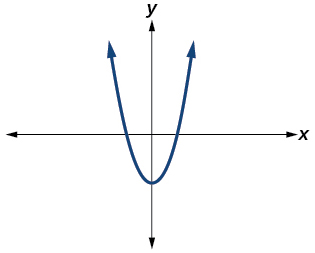2 real and 0 nonreal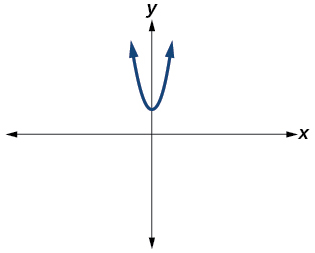For the following exercises, plot the complex numbers on the complex plane.

$1-2i$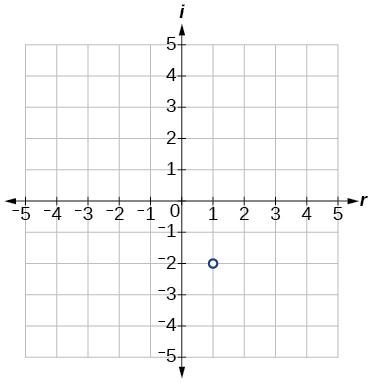$-2+3i$

$i$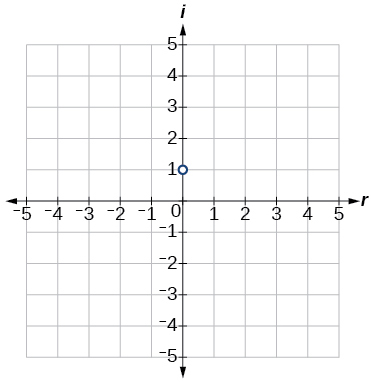$-3-4i$

## Numeric

For the following exercises, perform the indicated operation and express the result as a simplified complex number.

$\left(3+2i\right)+\left(5-3i\right)$

$8-i$

$\left(-2-4i\right)+\left(1+6i\right)$

$\left(-5+3i\right)-\left(6-i\right)$

$-11+4i$

$\left(2-3i\right)-\left(3+2i\right)$

$\left(-4+4i\right)-\left(-6+9i\right)$

$2-5i$

$\left(2+3i\right)\left(4i\right)$

$\left(5-2i\right)\left(3i\right)$

$6+15i$

$\left(6-2i\right)\left(5\right)$

$\left(-2+4i\right)\left(8\right)$

$-16+32i$

$\left(2+3i\right)\left(4-i\right)$

$\left(-1+2i\right)\left(-2+3i\right)$

$-4-7i$

$\left(4-2i\right)\left(4+2i\right)$

$\left(3+4i\right)\left(3-4i\right)$

25

$\frac{3+4i}{2}$

$\frac{6-2i}{3}$

$2-\frac{2}{3}i$

$\frac{-5+3i}{2i}$

$\frac{6+4i}{i}$

$4-6i$

$\frac{2-3i}{4+3i}$

$\frac{3+4i}{2-i}$

$\frac{2}{5}+\frac{11}{5}i$

$\frac{2+3i}{2-3i}$

$\sqrt{-9}+3\sqrt{-16}$

$15i$

$-\sqrt{-4}-4\sqrt{-25}$

$\frac{2+\sqrt{-12}}{2}$

$1+i\sqrt{3}$

$\frac{4+\sqrt{-20}}{2}$

${i}^{8}$

$1$

${i}^{15}$

${i}^{22}$

$-1$

## Technology

For the following exercises, use a calculator to help answer the questions.

Evaluate $\text{\hspace{0.17em}}{\left(1+i\right)}^{k}\text{\hspace{0.17em}}$ for Predict the value if $\text{\hspace{0.17em}}k=16.$

Evaluate $\text{\hspace{0.17em}}{\left(1-i\right)}^{k}\text{\hspace{0.17em}}$ for Predict the value if $\text{\hspace{0.17em}}k=14.$

128i

Evaluate $\text{\hspace{0.17em}}\left(1+i{\right)}^{k}-\left(1-i{\right)}^{k}$ for . Predict the value for $\text{\hspace{0.17em}}k=16.$

Show that a solution of $\text{\hspace{0.17em}}{x}^{6}+1=0\text{\hspace{0.17em}}$ is $\text{\hspace{0.17em}}\frac{\sqrt{3}}{2}+\frac{1}{2}i.$

${\left(\frac{\sqrt{3}}{2}+\frac{1}{2}i\right)}^{6}=-1$

Show that a solution of $\text{\hspace{0.17em}}{x}^{8}-1=0\text{\hspace{0.17em}}$ is $\text{\hspace{0.17em}}\frac{\sqrt{2}}{2}+\frac{\sqrt{2}}{2}i.$

## Extensions

For the following exercises, evaluate the expressions, writing the result as a simplified complex number.

$\frac{1}{i}+\frac{4}{{i}^{3}}$

$3i$

$\frac{1}{{i}^{11}}-\frac{1}{{i}^{21}}$

${i}^{7}\left(1+{i}^{2}\right)$

0

${i}^{-3}+5{i}^{7}$

$\frac{\left(2+i\right)\left(4-2i\right)}{\left(1+i\right)}$

5 – 5i

$\frac{\left(1+3i\right)\left(2-4i\right)}{\left(1+2i\right)}$

$\frac{{\left(3+i\right)}^{2}}{{\left(1+2i\right)}^{2}}$

$-2i$

$\frac{3+2i}{2+i}+\left(4+3i\right)$

$\frac{4+i}{i}+\frac{3-4i}{1-i}$

$\frac{9}{2}-\frac{9}{2}i$

$\frac{3+2i}{1+2i}-\frac{2-3i}{3+i}$

how fast can i understand functions without much difficulty
what is set?
a colony of bacteria is growing exponentially doubling in size every 100 minutes. how much minutes will it take for the colony of bacteria to triple in size
I got 300 minutes. is it right?
Patience
no. should be about 150 minutes.
Jason
It should be 158.5 minutes.
Mr
ok, thanks
Patience
100•3=300 300=50•2^x 6=2^x x=log_2(6) =2.5849625 so, 300=50•2^2.5849625 and, so, the # of bacteria will double every (100•2.5849625) = 258.49625 minutes
Thomas
what is the importance knowing the graph of circular functions?
can get some help basic precalculus
What do you need help with?
Andrew
how to convert general to standard form with not perfect trinomial
can get some help inverse function
ismail
Rectangle coordinate
how to find for x
it depends on the equation
Robert
yeah, it does. why do we attempt to gain all of them one side or the other?
Melissa
whats a domain
The domain of a function is the set of all input on which the function is defined. For example all real numbers are the Domain of any Polynomial function.
Spiro
Spiro; thanks for putting it out there like that, 😁
Melissa
foci (–7,–17) and (–7,17), the absolute value of the differenceof the distances of any point from the foci is 24.
difference between calculus and pre calculus?
give me an example of a problem so that I can practice answering
x³+y³+z³=42
Robert
dont forget the cube in each variable ;)
Robert
of she solves that, well ... then she has a lot of computational force under her command ....
Walter
what is a function?
I want to learn about the law of exponent
explain this

#### Get Jobilize Job Search Mobile App in your pocket Now!ByBy OpenStaxBy Carly AllenBy Jonathan LongBy Jessica CollettBy Rohini AjayBy Rohini AjayBy Madison ChristianBy Laurence BailenBy OpenStaxBy Jesenia Wofford